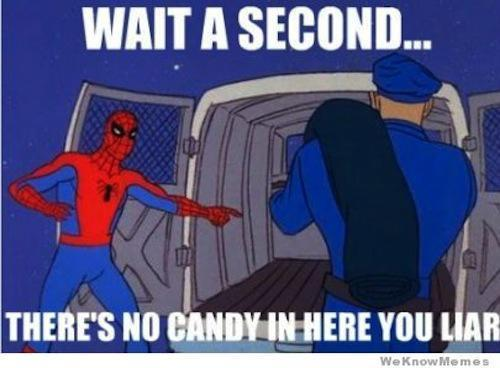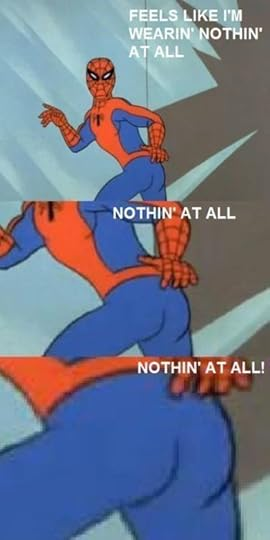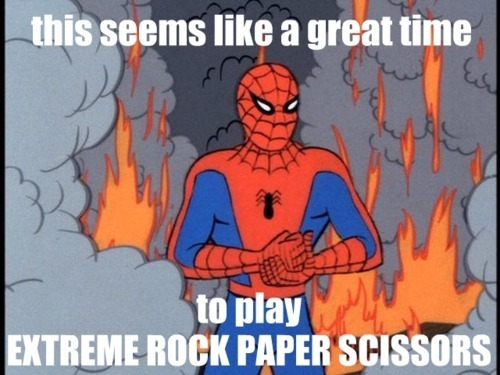# Just for fun discussion

3 views
FUNNIEST PICTURES EVER

FUNNY PICTURES

message 2: by Celine☆, HEAD MINION (new)

YAAAAAAAAAY

message 3: by Celine☆, HEAD MINION (last edited Dec 19, 2012 10:26AM) (new)message 7: by Celine☆, HEAD MINION (new)

XD XD XD XD XD XD LOVE IT

another one

message 12: by Celine☆, HEAD MINION (new)

Ive seen that one! CUTIE!

message 14: by Celine☆, HEAD MINION (new)

PEWW PEWW :D :D :D :D :D

litter box theif!

i am accusing you!

Of stealing crap!

message 21: by Celine☆, HEAD MINION (new)

HAHAHAHAHAHHA WELL DEDUCED, DETECTIVE KITTY.

Now i will bring in some witnesses to testify

message 26: by Celine☆, HEAD MINION (new)

ROFL GUILTY!

:D :D :D :D :D :D :D :D :D :D :D :D :D :D :D :D :D :D :D :D :D :D :D :D :D :D :D :D :D :D :D :D :D :D :D :D :D :D :D :D :D :D :D :D :D :D :D :D :D :D :D :D :D :D :D :D :D :D :D :D :D :D :D :D :D :D :D :D :D :D :D :D :D :D :D :D :D :D :D :D :D :D :D :D :D :D :D :D :D :D :D :D :D :D :D :D :D :D :D :D :D :D :D :D :D :D :D :D :D :D :D :D :D :D :D :D :D :D :D :D :D :D :D :D :D :D :D :D :D :D :D :D :D :D :D :D :D :D :D :D :D :D :D :D :D :D :D :D :D :D :D :D :D :D :D :D :D :D :D :D :D :D :D :D :D :D :D :D :D :D :D :D :D :D :D :D :D :D :D :D :D :D :D :D :D :D :D :D :D :D :D :D :D :D :D :D :D :D :D :D :D :D :D :D :D :D :D :D :D :D :D :D :D :D :D :D :D :D :D :D :D :D :D :D :D :D :D :D :D :D :D :D :D :D :D :D :D :D :D :D :D :D :D :D :D :D :D :D :D :D :D :D :D :D :D :D :D :D :D :D :D :D :D :D :D :D :D :D :D :D :D :D :D :D :D :D :D :D :D :D :D :D :D :D :D :D :D :D :D :D :D :D :D :D :D :D :D :D :D :D :D :D :D :D :D :D :D :D :D :D :D :D :D :D :D :D :D :D :D :D :D :D :D :D :D :D :D :D :D :D :D :D :D :D :D :D :D :D :D :D :D :D :D :D :D :D :D :D :D :D :D :D :D :D :D :D :D :D :D :D :D :D :D :D :D :D :D :D :D :D :D :D :D :D :D :D :D :D :D :D :D :D :D :D :D :D :D :D :D :D :D :D :D :D :D :D :D :D :D :D :D :D :D :D :D :D :D :D :D :D :D :D :D :D :D :D :D :D :D :D :D :D :D :D :D :D :D :D :D :D :D :D :D :D :D :D :D :D :D :D :D :D :D :D :D :D :D :D :D :D :D :D :D :D :D :D :D :D :D :D :D :D :D :D :D :D :D :D :D :D :D :D :D :D :D :D :D :D :D :D :D :D :D :D :D :D :D :D :D :D :D :D :D :D :D :D :D :D :D :D :D :D :D :D :D :D :D :D :D :D :D :D :D :D :D :D :D :D :D :D :D :D :D :D :D :D :D :D :D :D :D :D :D :D :D :D :D :D :D :D :D :D :D :D :D :D :D :D :D :D :D :D :D :D :D :D :D :D :D :D :D :D :D :D :D :D :D :D :D :D :D :D :D :D :D :D :D :D :D :D :D :D :D :D :D :D :D :D :D :D :D :D :D :D :D :D :D :D :D :D :D :D :D :D :D :D :D :D :D :D :D :D :D :D :D :D :D :D :D :D :D :D :D :D :D :D :D :D :D :D . OFFICERS! ARREST HER! *arrest celine* (go to jail topic)

message 28: by Celine☆, HEAD MINION (new)

*GASPING* NoOoOoOoOoOoOoOoOoOoOoOoOoOoOoOoOoOoOoOoOoOoOoOoOoOoOoOoOoOoOoOoOoOoOoOoOoOoOoOoOoOoOoOoOoOoOoOoOoOoOoOoOoOoOoOoOoOoOoOoOoOoOoOoOoOoOoOoOoOoOoOoOoOoOoOoOoOoOoOoOoOoOoOoOoOoOoOoOoOoOoOoOoOoOoOoOoOoOoOoOoOoOoOoOoOoOoOoOoOoOoOoOoOoOoOoOoOoOoOoOoOoOoOoOoOoOoOoOoOoOoOoOoOoOoOoOoOoOoOoOoOoOoOoOoOoOoOoOoOoOoOoOoOoOoOoOoOoOoOoOoOoOoOoOoOoOoOoOoOoOoOoOoOoOoOoOoOoOoOoOoOoOoOoOoOoOoOoOoOoOoOoOoOoOoOoOoOoOoOoOoOoOoOoOoOoOoOoOoOoOoOoOoOoOoOoOoOoOoOoOoOoOoOoOoOoOoOoOoOoOoOoOoOoOoOoOoOoOoOoOoOoOoOoOoOoOoOoOoOoOoOoOoOoOoOoOoOoOoOoOoOoOoOoOoOoOoOoOoOoOoOoOoOoOoOoOoOoOoOoOoOoOoOoOoOoOoOoOoOoOoOoOoOoOoOoOoOoOoOoOoOoOoOoOoOoOoOoOoOoOoOoOoOoOoOoOoOoOoOoO!!!!!!!!!!!!!

message 29: by [deleted user] (new)

ACHTUNG!

message 30: by [deleted user] (new)

means stop i think

message 31: by [deleted user] (new)

in german

message 32: by Celine☆, HEAD MINION (new)

Oh. Gold star. ^.^